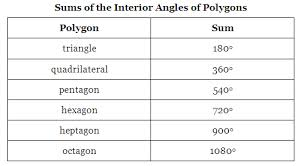Mathematic

# Discuss on Angle Sum of PolygonsPrime objective of this assignment is to Discuss on Angle Sum of Polygons. Here explain Angle Sum of Polygons in mathematical point of view. The interior angle sum of this polygon can now be found by multiplying the quantity of triangles by 180°. On analysis, it is found that the quantity of triangles is always two less than the quantity of sides. This fact is stated as being a theorem.